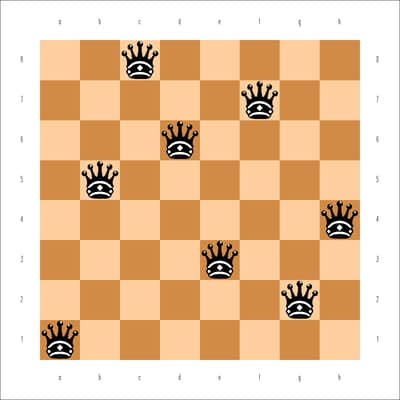### Eight Queens

The Eight Queens problem is a special instance of the
-Queens problem, where the objective is to position
queens on an×chessboard such that no two queens attack each other. The CLP procedure provides an expressive constraint for variable arrays that can be used for solving this problem very efficiently.

You can model this problem by using a variable array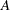of dimension, where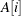is the row number of the queen in column. Since no two queens can be in the same row, it follows that all the’s must be pairwise distinct.

In order to ensure that no two queens can be on the same diagonal, the following should be true for alland: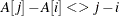and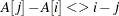In other words,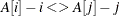and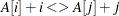Hence, the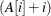’s are pairwise distinct, and the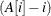’s are pairwise distinct.

These two conditions, in addition to the one requiring that the’s be pairwise distinct, can be formulated using the FOREACH statement.

One possible such CLP formulation is presented as follows:

```proc clp out=out
varselect=fifo; /* Variable Selection Strategy               */
array A (A1-A8);   /* Define the array A                        */
var (A1-A8)=[1,8];    /* Define each of the variables in the array */
/* Initialize domains                        */
/* A[i] is the row number of the queen in column i*/
foreach(A, DIFF,  0); /* A[i] 's are pairwise distinct */
foreach(A, DIFF, -1); /* A[i] - i 's are pairwise distinct */
foreach(A, DIFF,  1); /* A[i] + i 's are pairwise distinct */
run;
```

The ARRAY statement is required when you are using a FOREACH statement, and it defines the array A in terms of the eight variables A1–A8. The domain of each of these variables is explicitly specified in the VARIABLE statement to be the digits 1 through 8 since they represent the row number on an 8×8 board. FOREACH(A, DIFF, 0) represents the constraint that the’s are different. FOREACH(A, DIFF, –1) represents the constraint that the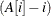’s are different, and FOREACH(A, DIFF, 1) represents the constraint that the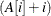’s are different. The VARSELECT= option specifies the variable selection strategy to be first-in-first-out, the order in which the variables are encountered by the CLP procedure.

The following statements display the solution data set shown in Figure 3.2:

```proc print data=out noobs label;
label A1=a A2=b A3=c A4=d
A5=e A6=f A7=g A8=h;
run;
```

Figure 3.2: A Solution to the Eight Queens Problem

a b c d e f g h
1 5 8 6 3 7 2 4

The corresponding solution to the Eight Queens problem is displayed in Figure 3.3.

Figure 3.3: A Solution to the Eight Queens Problem